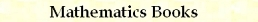Integer Numbers C/C++ Macros
by Stanislav Sýkora, Extra Byte, Via R.Sanzio 22C, Castano Primo, Italy 20022
in Stan's Library, Ed.S.Sykora, Vol.III. First release March 6, 2011
Permalink via DOI:  10.3247/SL4Soft11.001
 PREVIOUS | Other code snippets | Software Books and Links Programming Section of Stan' LIBRARY | Stan's HUB
 This document is part of a collection of macros. For introductory remarks, see the first part (General Purpose Macros). For a review of macro-syntax rules and related tips and tricks, see Writing Macros. For the typedefs DWORD, WORD, BYTE and CHAR click here.
 CATEGORY MACRO SIMPLE TESTS mIsOdd, mIsEven mIsNotMultiple, mIsMultiple, mIsModEqu mIsNot2ToN, mIs2ToN, mIsPowerOf2 CONVERSIONS mToggleEndian mInt2Gray, mGray2Int EVEN | ODD PARITY mLeEven, mGeEven, mLtEven, mGtEven mLeOdd, mGeOdd, mLtOdd, mGtOdd mBitsCount MODULO CALCULUS mModIndex mModLe, mModLt, mModGe, mModGt ARITHMETICS mSetLe2ToN, mSetLt2ToN, mSetGe2ToN, mSetGt2ToN
 Simple testsCode: #define mIsOdd(n) ((n)&1) #define mIsEven(n) (!IsOdd(n)) #define mIsNotMultiple(m,d) ((m)%(d)) #define mIsMultiple(m,d) (!mIsNotMultiple(m,d)) #define mIsModEqu(m,n,d) ((m)%(d)==(n)%(d)) #define mIsNot2ToN(n) ((n)&((n)-1)) #define mIs2ToN(n) (!mIsNot2ToN(n)) #define mIsPowerOf2(n) ((n)&& mIs2ToN(n)) Arguments: n, m, d are expressions of an integer type (or ones that can be cast to an integer type). Additional limitations (where they exist) are listed separately for each case. The return value is either true (1) or false (0). mIsOdd(n) tests whether n is an odd integer value. mIsEven(n) tests whether n is an even integer value divisible by 2. mIsMultiple(m,d), mIsNotMultiple(m,d) tests whether m is or is not a multiple of d or, equivalently, whether d is or is not a divisor of m. The arguments m, d must be either both signed or both unsigned integers. The width of d may be smaller than that of m; it should not be larger, though even that would in many cases work. mIsModEqu(m,n,d) tests whether the integer values m, n are equal modulo d. The arguments must be either all signed or all unsigned integers. mIsNot2ToN(n), mIs2ToN(n), mIsPowerOf2(n) tests whether an unsigned integer (or a signed one, but non-negative) n is a power of 2. These very efficient expressions exploit the fact that (n)&((n)-1) differs from (n) by the removal of the least-significant non-zero binary bit. For example, when (n) = bx01011000 then ((n)-1) = bx01010111 and (n)&((n)-1) = bx01010000. When n is a power of 2, it has only one binary bit set and (n)&((n)-1) evaluates to zero, while for all other positive numbers the result is non-zero. However, zero evaluates also to 0 because it gets interpreted as 2^W, where W is the width (8, 16, 32,... bits) of the argument. Hence, if 0 is not to be included among the powers of 2, a separate test needs to be done, such as in mIsPowerOf2(n).
 ConversionsCode: #define mSwapBytes(lpData,b1,b2) \    {BYTE b;mSwap(((BYTE*)(lpData))[b1],((BYTE*)(lpData))[b2],b);}   #define mToggleEndian(lpn) \    {DWORD i, B=sizeof(*(lpn))-1; for (i=0;i+i<=B;i++) {mSwapBytes(lpn,i,B-i);}}   #define mInt2Gray(n) ((n)^((n)>>1)) #define mGray2Int(g,lvn) {DWORD s=1; (lvn)=(g); while (true) \    {(lvn)^=(lvn)>>s; if (((lvn)>>s)>>s) s+=s; else break;}} Arguments: lpn is a pointer to an integer of any type (signed|unsigned) and width. n, g are unsigned integer expressions of any width lvn is a left-value integer expressions of any width The macro mSwapBytes(lpData,b1,b2) is an auxiliary used just to improve readability of mToggleEndian. Though it might be of a more general use, here it is intended locally, not to be called directly by Users. It swaps the byte with index b1 of the generic structure pointed to by lpData with its byte with index b2. Naturally, b1 and b2 must be non-negative integer expressions. mToggleEndian(lpn) toggles the integer pointed to by lpn (of any type and width) between the little-endian (least significant byte first) and the big-endian (most significant byte first) encoding. In this context, the term toggle means that a little-endian number is replaced by its big-endian version and vice-versa (the operation is an inverse of itself). This requires a byte-reversal of the B-bytes structure such that the last byte becomes the first one and so on. The conversion is done in-place, i.e., the result replaces the input. mInt2Gray(n) converts an unsigned integer n to the corresponding binary Gray code. This is a simple expression macro. mGray2Int(g,lvn) converts a Gray code g to the corresponding unsigned integer and sets the result into lvn. This is an optimized composite-statement macro using an auxiliary variable.
 Even/Odd ParityCode: #define mLeEven(n) ((n)&1?(n)-1:(n);) #define mGeEven(n) ((n)&1?(n)+1:(n);) #define mLtEven(n) ((n)&1?(n)-1:(n)-2;) #define mGtEven(n) ((n)&1?(n)+1:(n)+2;)   #define mLeOdd(n) ((n)&1?(n):(n)-1;) #define mGeOdd(n) ((n)&1?(n):(n)+1;) #define mLtOdd(n) ((n)&1?(n)-2:(n)-1;) #define mGtOdd(n) ((n)&1?(n)+2:(n)+1;)   #define mBitsCount(nn,lvc) {DWORD temp=(nn); (lvc)=0; while(temp){(lvc)++;temp&=temp-1;}} Arguments: n is an integer expression (signed or unsigned). nn is an unsigned integer expression lvc is a non-negative integer left-value Parity has a dual meaning: either even/odd integer value, or even/odd number of binary bits set in a non-negative integer Macros pertinent to both cases are covered in this category. mLeEven(n), mGeEven(n), mLtEven(n),mGtEven(n) returns the nearest even value which is respectively ≤, ≥, < or > n. mLeOdd(lvn), mGeOdd(lvn), mLtOdd(lvn), mGtOdd(lvn) returns the nearest odd value which is respectively ≤, ≥, < or > n. mBitsCount(nn,lvc), expression macro setting in the counter lvc the number of binary bits which are set in nn.
 Modulo CalculusCode: #define mModIndex(n,d) ((n)%(d))   #define mModLe(n,d) ((n)-((n)%(d))) #define mModGe(n,d) ((n)%(d)?(n)+(d)-((n)%(d)):(n)) #define mModLt(n,d) ((n)%(d)?(n)-((n)%(d)):(n)-(d)) #define mModGt(n,d) ((n)+(d)-((n)%(d))) Arguments: n is an integer expression (or one that can be cast to an integer type). d is a positive expression of an integer type (or one that can be cast to an integer type). mModIndex(n,d) returns n % d, which is the index of n in modulo-d calculus. mModLe(n,d), mModGe(n,d), mModLt(n,d),mModGt(n,d) returns the nearest modulo-d boundary which is ≤, ≥, < or > n.
 ArithmeticsCode: #define mSetLe2ToN(lvn) {while (mIsNot2ToN(lvn)) (lvn)&=(lvn)-1;} #define mSetGe2ToN(lvn) {if (mIsNot2ToN(lvn)) {mSetLe2ToN(lvn);(lvn)<<=1;}} #define mSetLt2ToN(lvn) {if (mIsNot2ToN(lvn)) mSetLe2ToN(lvn); else (lvn)>>=1;} #define mSetGt2ToN(lvn) {mSetLe2ToN(lvn);(lvn)<<=1;} Arguments: lvn is an unsigned, positive integer left-value. mSetLe2ToN(lvn), mSetGe2ToN(lvn), mSetLt2ToN(lvn), mSetGt2ToN(lvn), replaces lvn with the nearest power of 2 which is ≤, ≥, < or > lvnTOP | PREVIOUS | NEXT | Other code snippets | Software Books and Links Programming Section of Stan' LIBRARY | Stan's HUB
 Copyright ©2011 Stanislav Sýkora    DOI: 10.3247/SL4Soft11.001 Designed by Stan Sýkora